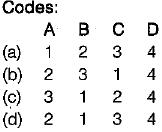Courses

# Test: Points And Crossings

## 10 Questions MCQ Test Topicwise Question Bank for GATE Civil Engineering | Test: Points And Crossings

Description
This mock test of Test: Points And Crossings for Civil Engineering (CE) helps you for every Civil Engineering (CE) entrance exam. This contains 10 Multiple Choice Questions for Civil Engineering (CE) Test: Points And Crossings (mcq) to study with solutions a complete question bank. The solved questions answers in this Test: Points And Crossings quiz give you a good mix of easy questions and tough questions. Civil Engineering (CE) students definitely take this Test: Points And Crossings exercise for a better result in the exam. You can find other Test: Points And Crossings extra questions, long questions & short questions for Civil Engineering (CE) on EduRev as well by searching above.
QUESTION: 1

### Match List-I and List-ll and select correct answer using codes given below the lists: List-1 A. Distance between adjoining face of running rail and check rail B. Distance through which the tongue rail moves laterally at the toe of switch for the movement of rails C. Distance between the gauge faces of the stock rail and tongue rail at the heel D. Angle between the gauge face of stock rail and tongue rail List-II 1. Heel divergence 2. Flangeway clearance 3. Throw of switch 4. Switch angleSolution:

Flangeway clearance: Distance between adjoining face of running rail and check rail.
Throw of switch: It is the distance through which the toe of the tongue moves sideways to provide a path for the desired direction over the turnout

QUESTION: 2

### If α is the angle of crossing, then the number of crossing ‘N’ according to right angle method is given by

Solution:

Answer (b) and (d) are correct for centre line method and isosceles trianale method respectively.

QUESTION: 3

### Lead of crossing is the distance from the

Solution:
QUESTION: 4

Number of switches provided on a Gauntletted track is

Solution:
QUESTION: 5

The correct relation between Curve Lead (CL), Switch Lead (SL) and Lead of crossing (L) is given by

Solution:
QUESTION: 6

In a scissors crossover, the crossing provided are
(i) 2 obtuse angle crossings
(ii) 4 obtuse angle crossings
(iii) 4 acute angle crossings
(iv) 6 acute angle crossings

The correct answer is

Solution:
QUESTION: 7

The distance through which the tongue rail moves laterally at the toe of the switch for movement of trains is called

Solution:
QUESTION: 8

Flangeway clearance is the distance

Solution:
QUESTION: 9

Heel divergence is

Solution:

Heel divergence is equal to flange way clearance plus tolerance for the wear plus the width of head of rail.

QUESTION: 10

If 'A’ is the angle formed by two gauge faces, the crossing number will be

Solution: